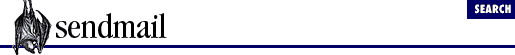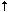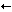[Chapter 6] 6.3 The local Delivery Agent

#Chapter 6The Mail Hub and Delivery Agents## 6.3 The local Delivery Agent

When you ran sendmail, it complained that the `local` delivery agent definition was missing. To keep sendmail happy, this definition will now be added to the client.cf file. As it happens, it is already in your system sendmail.cf file (if you have one), and you can copy it by typing the following command:

`% `grep "^Mlocal" /etc/sendmail.cf >> client.cf``

Note that the `^M` above is actually two characters `^` and `M`, not a CTRL-M. Now load the client.cf file into your editor. It will look something like this: 

 If any of the equates shown here are missing, you need to type them in by hand.

```# This is a comment
V7      # this is another comment
# Delivery agent definition to forward mail to hub
Mhub, P=[IPC], A=IPC \$h
`Mlocal, P=/bin/mail, F=lsDFMAw5:/|@rmn, S=10, R=20/40, A=mail -d \$u````

Right off the bat, you'll notice three new equates  that are a bit more complicated than our original `hub` equates. This new `M` configuration command declares a symbolic name, such as `hub`. Here, that name is `local` - the name that sendmail complained was missing. Although the `local` definition is important and heavily used in a full-fledged sendmail.cf file, the client.cf file uses it only to keep sendmail from complaining.

 You may also find a `T=` equate. We describe it later in this chapter.

The new delivery agent definition is composed of six parts (each separated from the others by commas), a symbolic name and six equates. The `F=`, `S=`, and `R=` equates are new. You've seen the `M`, `P=`, and `A=` before in the `hub` definition.

`M`

All mail delivery agent definitions begin with the `M` configuration-file command. Like all configuration commands, that `M` must begin a line:

````Mhub`,   P=[IPC], A=IPC \$h
`Mlocal`, P=/bin/mail, F=lsDFMAw5:/|@rmn, S=10, R=20/40, A=mail -d \$udefine a delivery agent```

The symbolic name for each delivery agent follows the `M`, with no intervening space. The symbolic names here are `hub` and `local`. The delivery agent called `hub` forwards mail to the central hub machine. The `local` delivery agent delivers mail to users on the local machine.

`P=`

The `P=` equate (for Path) specifies the full pathname of mail delivery programs:

```Mhub,   `P=[IPC]`, A=IPC \$h
Mlocal, `P=/bin/mail`, F=lsDFMAw5:/|@rmn, S=10, R=20/40, A=mail -d \$ufull pathname of program```

Your program names may differ, but in general, the program for `local` places a mail message into the local user's mail spool file.

`A=`

The `A=` equate (for Argument vector) specifies the command-line arguments to be supplied to each mail delivery program.

```Mhub,   P=[IPC], `A=IPC \$h`
Mlocal, P=/bin/mail, F=lsDFMAw5:/|@rmn, S=10, R=20/40, `A=mail -d \$u `command line (argv)```

Notice that `local` uses the `\$u` macro, whereas `hub` uses the `\$h` macro. The `\$u` macro contains the name of the recipient (such as bob). The `\$h` macro contains the name of a host (such as here.us.edu). Macros are explained in the next chapter. By convention the `A=` equate is usually last.

Three parts in the new `local` definition were not used in the `hub` definition. They are:

`F=`

The `F=` equate (for Flags) specifies certain flags that tell sendmail more about the delivery agent. Each flag is a single character, and each is Boolean - either set (if it is present) or not (if it is absent).

```Mhub,   P=[IPC], A=IPC \$h
Mlocal, P=/bin/mail, `F=lsDFMAw5:/|@rmn`, S=10, R=20/40, A=mail -d \$uflags for delivery agent```

There are many flags to choose from. They are all described in Chapter 30, Delivery Agents, but we will cover a few of them later in this chapter.

`S=`

The `S=` equate (for Sender) specifies which rule set to use in rewriting the sender's address:

```Mhub,   P=[IPC], A=IPC \$h
Mlocal, P=/bin/mail, F=lsDFMAw5:/|@rmn, `S=10`, R=20/40, A=mail -d \$usender rule set```

Addresses need to be rewritten because different delivery agents require addresses to be in different forms. For example, the `[IPC]` agent requires the form [email protected], while the `uucp` agent (if you had need for one) requires the form host!user. Here, the `S=` says that addresses should be rewritten by using rule set 10. We will cover rule sets later, in Chapter 8, Addresses and Rules.

`R=`

The `R=` equate (for Recipient) specifies which rule set to use in rewriting the recipient's address:

```Mhub,   P=[IPC], A=IPC \$h
Mlocal, P=/bin/mail, F=lsDFMAw5:/|@rmn, S=10, `R=20/40`, A=mail -d \$urecipient rule set```

Again, those addresses need to be rewritten because different delivery agents require different forms of addresses. Here, the `R=` says to use rule set 20 in rewriting an envelope address and rule set 40 in rewriting a header address.

In addition to the three new equates, and beginning with V8.7 sendmail, you may discover another new equate in the `local` delivery agent definition:

`T=`

Not shown in our examples is the `T=` equate (for Type), which lists three fields of information about the delivery agent. You will find this equate in configuration files beginning with V8.7 sendmail.

`Mlocal, ..., `T=DNS/RFC822/X-Unix``

First is the type of MTA used (here `DNS` because sendmail is using DNS to lookup addresses); then a slash followed by the type of addressing used (here `RFC822`, but it could also be, for example, `X.400`); and last a slash followed by the type of error messages produced (here `X-Unix` which says that the program /bin/mail will produce UNIX errors). The `T=` equate is used to support Delivery Status Notification (DSN) as described in RFC1891, RFC1892, RFC1893, and RFC1894.

### 6.3.1 Skipping Rule Sets

Because we won't be covering rule sets for a while, and to simplify things for now, edit the client.cf file once again and change the `S=` and `R=` equates in the new `local` definition:

```# Mailer to forward all mail to the hub machine
Mhub,   P=[IPC], A=IPC \$h
Mlocal, P=/bin/mail, F=lsDFMAw5:/|@rmn, `S=0, R=0`, A=mail -d \$unew     new```

Both equates are changed to zero because there are no rule sets yet. When an `S=` or an `R=` equate in a delivery agent definition is zero or missing, sendmail skips the delivery-agent-specific part of rule-set processing.

Comments are an important part of every configuration file. They remind you of what you are trying to do now and what you have done in the past. Edit the client.cf file now. Remove two old comments and add a new one:

```removed comment
V7removed comment
# Delivery agent definition to forward mail to hub
Mhub,   P=[IPC], A=IPC \$h
`# Sendmail requires this, but we won't use it.      `added comment
Mlocal, P=/bin/mail, F=lsDFMAw5:/|@rmn, S=0, R=0, A=mail -d \$u```

We threw away the earlier comments because they were only for demonstration purposes.

### 6.3.3 Testing the New Delivery Agent Definitions

This time, run sendmail differently than you did last time:

`% `/usr/lib/sendmail -d0.15 -Cclient.cf -bt </dev/null``

The `-d0.15` debugging switch tells sendmail (among other things) to show you how it interpreted your delivery agent definitions. The `-bt` causes sendmail to run in rule-testing mode so that the delivery agents will be displayed. Running the above command line produces output like the following (but with long lines unattractively wrapped at the right-hand margin):

```assorted other information here and above
mailer 0 (prog): P=/bin/sh S=0/0 R=0/0 M=0 U=0:0 F=Dlos L=0 E=\n T=DNS/RFC822/X-U
nix A=sh -c \$u
mailer 1 (*file*): P=[FILE] S=0/0 R=0/0 M=0 U=0:0 F=DEFMPlosu L=0 E=\n T=DNS/RFC8
22/X-Unix A=FILE
mailer 2 (*include*): P=/dev/null S=0/0 R=0/0 M=0 U=0:0 F=su L=0 E=\n T=<undefine
d>/<undefined>/<undefined> A=INCLUDE
mailer 3 (hub): P=[IPC] S=0/0 R=0/0 M=0 U=0:0 F= L=0 E=\n T=<undefined>/<undefine
d>/<undefined> A=IPC \$h
mailer 4 (local): P=/bin/mail S=0/0 R=0/0 M=0 U=0:0 F=/5:@ADFMlmnrsw| L=0 E=\n T=
<undefined>/<undefined>/<undefined> A=mail -d \$u```

This output, in addition to verifying that sendmail properly interpreted the client.cf file, reveals four equates you haven't seen before: `M=`, `U=`, `L=`, and `E=`. We won't explain them here, because you don't need them for the client.cf file. They are explained in detail in Chapter 30.

In the preceding output, also note that there are several equates that were not included in the original `Mhub` delivery agent definition. The `hub` definition included only the `P=` and `A=` equates:

`Mhub,   P=[IPC], A=IPC \$h`

When sendmail saw this definition, it did not find specifications for any of the equates other than `A=` and `P=`. Rather than complaining, it gave `E=` the value of the newline character (`\n`), `T=` three instances of `<undefined>`, and the other equates each a value of zero:

```mailer 3 (hub): P=[IPC] S=0/0 R=0/0 M=0 U=0:0 F= L=0 E=\n T=<undefined>/<undefine
d>/<undefined> A=IPC \$h```

Note that when the `F=` equate has a zero value, it is displayed as an empty list of flags.6.2 Define a Mail Delivery Agent6.4 Add the Missing Parts to Mhub